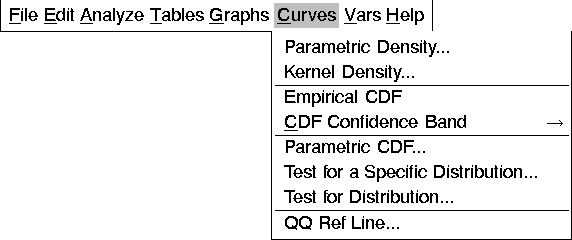Distribution Analyses

# Curves

Density estimation is the construction of an estimate of the density function from the observed data. The methods provided for univariate density estimation include parametric estimators and kernel estimators.

Cumulative distribution analyses include the empirical and the parametric cumulative distribution function. The empirical distribution function is a nonparametric estimator of the cumulative distribution function. You can fit parametric distribution functions if the data are from a known family of distributions, such as the normal, lognormal, exponential, or Weibull.

You can use the Kolmogorov statistic to construct a confidence band for the unknown distribution function. The statistic also tests the hypotheses that the data are from a completely specified distribution or from a specified family of distributions with unknown parameters.

You can generate density estimates and cumulative distribution analysis in the output options dialog, as described previously in the section "Output," or by choosing from the Curves menu, as shown in Figure 38.22. You can also generate QQ reference lines from the Curves menu.If you select a Weight variable, curves of parametric weighted normal density, weighted kernel density, weighted empirical CDF, parametric weighted normal CDF, and weighted QQ reference line (based on weighted least squares) can be generated. CDF confidence band, test for a specific distribution, and test for distribution are not computed.